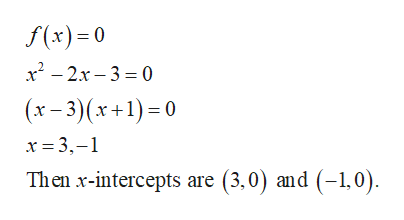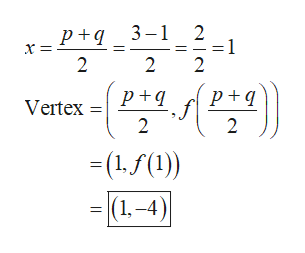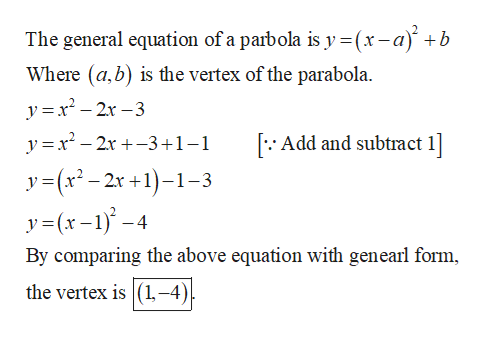# Given the parabola f(x)= x2-2x-3 complete parts (1) through (3) below. Find the vertex by averaging the x-intercepts.Find the vertex by completing the square.Find the vertex of f(x)= x2+5x+2 using your method of choice.What are the domain and range for f(x)=x2+5x+2?

Question
17 views

Given the parabola f(x)= x2-2x-3 complete parts (1) through (3) below.

1. Find the vertex by averaging the x-intercepts.

2. Find the vertex by completing the square.

3. Find the vertex of f(x)= x2+5x+2 using your method of choice.

4. What are the domain and range for f(x)=x2+5x+2?

check_circle

Step 1
1. Obtain the vertex by averaging the x-intercept.

Equate f (x) to 0 and obtain the x-intercepts.help_outlineImage Transcriptionclosef(x)= 0 x - 2x – 3 = 0 (x – 3)(x+1)=0 x = 3,-1 Then x-intercepts are (3,0) and (-1,0). fullscreen
Step 2

Consider p = 3 and q = –1 and the vertex can be obtaining by axis of symmetry.help_outlineImage Transcriptionclosep+q_ 3-1 2 =1 3 -1 2 p+q p+q Vertex =(1.f(1)) )| (1, –4) fullscreen
Step 3
1. Find the vertex by completing the ...help_outlineImage TranscriptioncloseThe general equation of a parbola is y =(x-a)' +b Where (a,b) is the vertex of the parabola. y =x² – 2x – 3 y =x – 2x +-3+1-1 [:: Add and subtract 1] y = (x² – 2r +1)–1-3 y=(x-1) -4 2.x By comparing the above equation with genearl form, the vertex is (1,–4)| fullscreen

### Want to see the full answer?

See Solution

#### Want to see this answer and more?

Solutions are written by subject experts who are available 24/7. Questions are typically answered within 1 hour.*

See Solution
*Response times may vary by subject and question.
Tagged in

### Applications of Mathematics